Function Repository Resource:

# Pyritohedron

Create a pyritohedron

Contributed by: Jan Mangaldan
 ResourceFunction["Pyritohedron"][h] returns a pyritohedron with displacement parameter h, represented as a Polyhedron object.

## Details

h is usually taken to be between 0 and 1.
A pyritohedron is a dodecahedron with pyritohedral symmetry (point group Th in Schönflies notation).
ResourceFunction["Pyritohedron"][] is equivalent to ResourceFunction["Pyritohedron"][1/2]. This corresponds to an idealized version of a crystal of the mineral pyrite.

## Examples

### Basic Examples (1)

A pyritohedron:

 In:=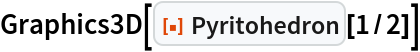Out=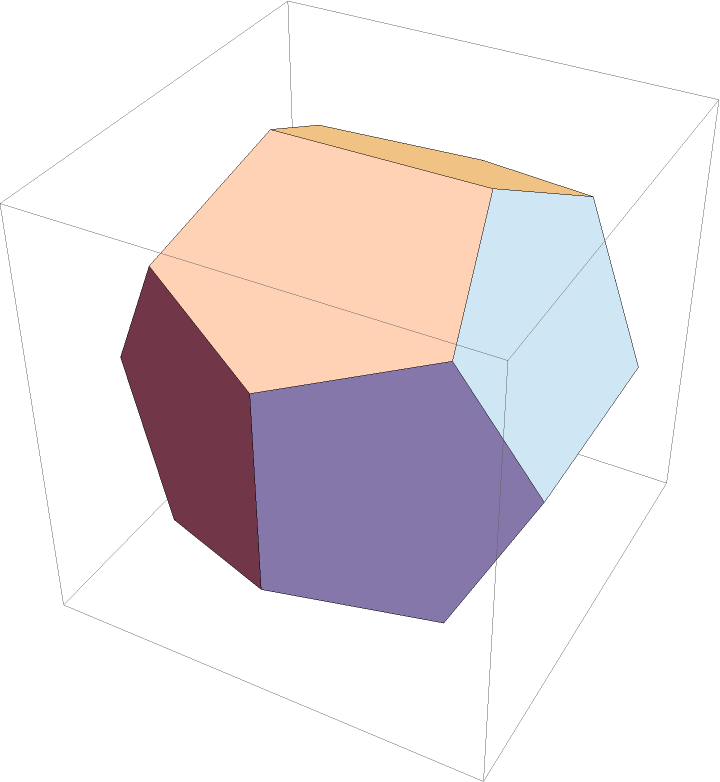### Applications (2)

Surface area and volume of a pyritohedron:

 In:=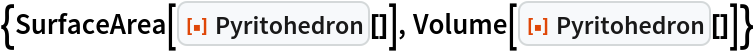Out=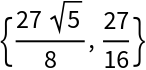Moment of inertia matrix for a pyritohedron:

 In:=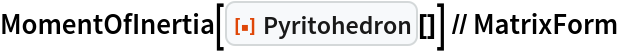Out=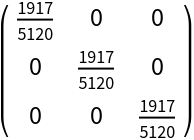### Properties and Relations (3)

Pyritohedron[] is equivalent to Pyritohedron[1/2]:

 In:=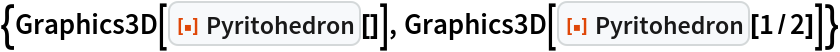Out=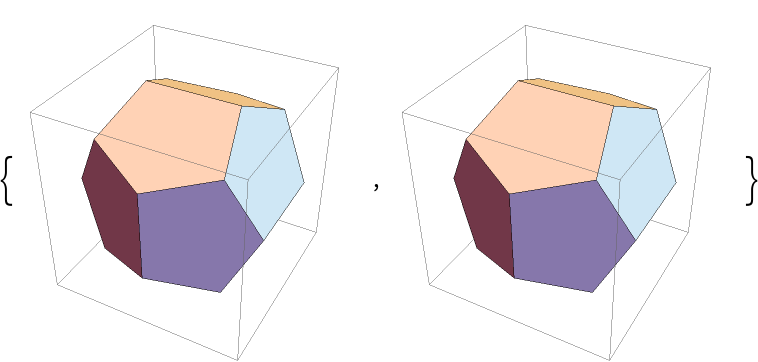Pyritohedron[1/GoldenRatio] is equivalent to a regular dodecahedron:

 In:=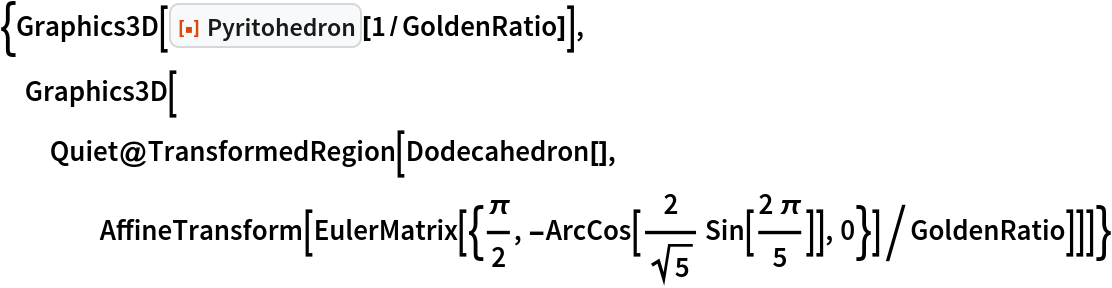Out=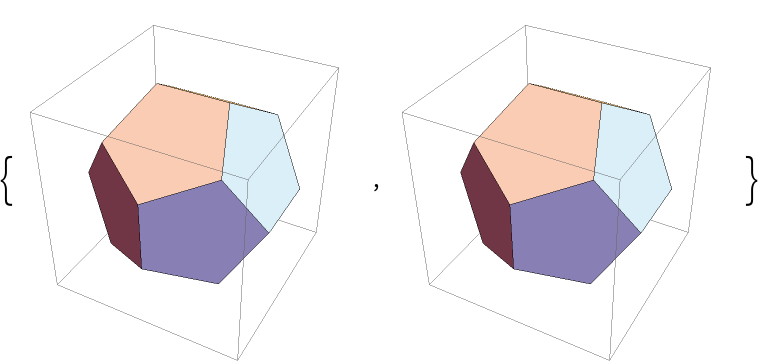A pyritohedron is self-dual:

 In:=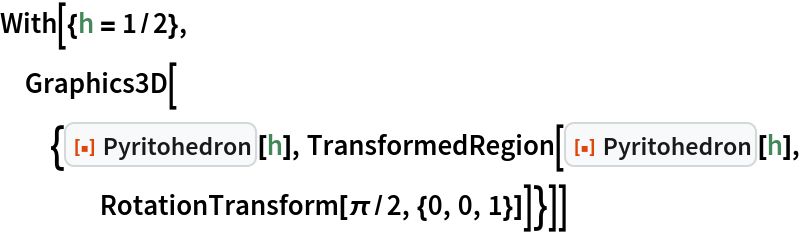Out=### Neat Examples (1)

Show the effect of varying the displacement:

 In:=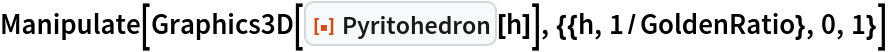Out=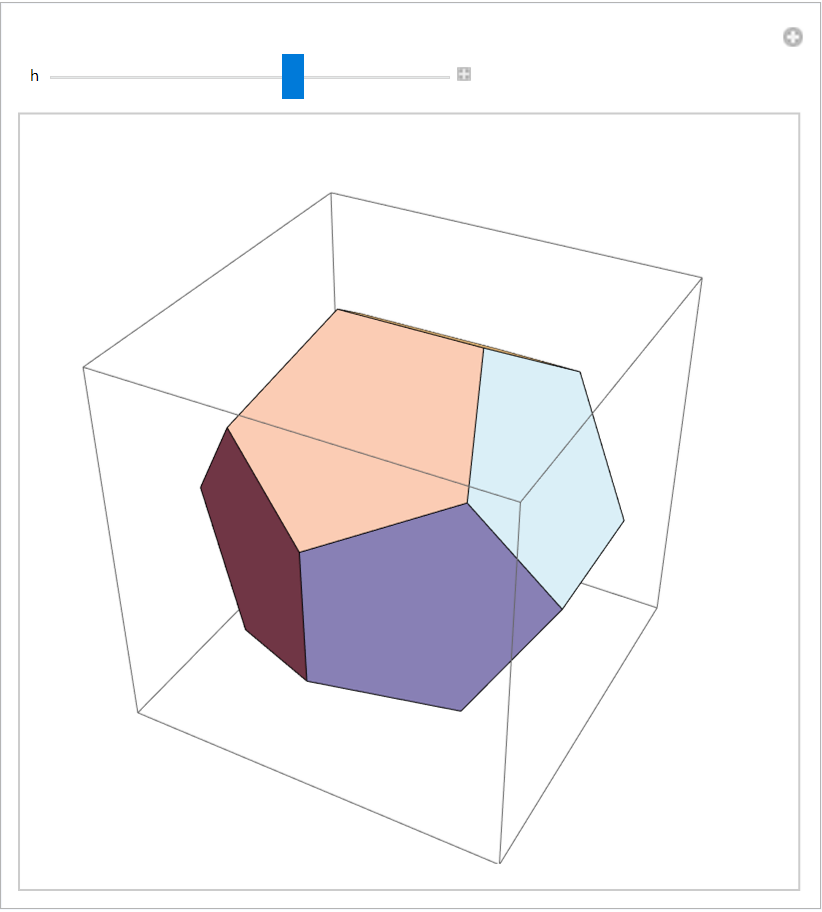## Version History

• 1.0.0 – 07 July 2021